# High School Chemistry : Identifying Reaction Equilibrium

## Example Questions

### Example Question #31 : Chemical Equilibrium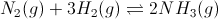Figure 1: Ammonia gas formation and equilibrium

What would most likely happen if a scientist decreased the volume of the container in which the reaction occurs?

Possible Answers:

More H2 would form

More N2 would form

More NH3 would form

Less NH3 would form

A violent explosion would occur

Correct answer:

More NH3 would form

Explanation:

Le Chatelier's principle states that changes in pressure are attributable to changes in volume. If we increase the volume, the reaction will shift toward the side that has more moles of gas. If we decrease the volume, the reaction will shift toward the side that has less moles of gas. Since the product side has only two moles of gas, compared to the reactant side with four moles, the reaction would shift toward the product side, and more NH3 would form.

### Example Question #1 : Identifying Reaction Equilibrium

Which of the answer choices correctly identifies chemical equilibrium?

Possible Answers:

The products have all been used up in further reactions

The reactants have all been converted to products

The products have all been converted to reactants

The forward and reverse reactions are occurring at the same rate

The concentration of reactants and concentration of products are equal

Correct answer:

The forward and reverse reactions are occurring at the same rate

Explanation:

Chemical equilibrium is defined as a state when the rates of the forward and reverse reactions are equal. Different reactions will have different concentrations of products are reactants, and concentration can vary depending on the process. Chemical equilibrium, however, is always the point at which there is no bias towards creating products or reactants.

If the forward reaction rate and reverse reaction rate are equal, then there is no net change in concentration of the reactants or products. This makes them appear to be stable.

### Example Question #15 : Equilibrium

When a reaction is at equilibrium __________.

Possible Answers:

the forward reaction rate is equal to zero

the forward reaction rate is equal to the reverse reaction rate

the reverse reaction rate is equal to zero

The forward reaction rate is slower than the reverse reaction rate

Correct answer:

the forward reaction rate is equal to the reverse reaction rate

Explanation:

When a reaction is at equilibrium, the concentrations of reactants and products are no longer increasing or decreasing; however, this does not mean that the forward and reverse reactions have stopped taking place. They are simply taking place at the same rate so that the concentrations of products and reactants do not change. Equilibrium is a dynamic process. Reactions are still taking place, but they offset one another in terms of rate. Essentially, every product that is created is immediately converted back to reactant by the reverse process, making the concentration of reactants and products appear constant.

### Example Question #16 : Equilibrium

Consider the following balanced reaction: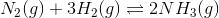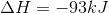What will increasing the pressure do to the system?

Possible Answers:

It will shift to the right

Nothing will happen

It depends on the temperature of the system

It will shift to the left

Correct answer:

It will shift to the right

Explanation:

Le Chatelier's principle states that a system will shift in a particular direction in order to reduce introduced stress to the system. In other words, if something is added to one side of the equation, the other side will consequently increase in order to restore equilibrium.

When gases are involved in the reaction, pressure increases on the side of the reaction with more moles of gas. Adding an additional amount of pressure will push the reaction toward the side with fewer moles of gas. This helps lower the pressure inside the container.There are four moles of gas on the reactants side, and two moles on the products side. If pressure is increased, the system will shift to the right in order to adjust to the new pressure.

### Example Question #21 : Chemical Reactions

Consider the following balanced reaction: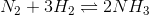When the reaction is at equilibrium, which of the following actions will result in more products?

Possible Answers:

Lower the temperature

Remove nitrogen gas

Remove hydrogen gas

Increase temperature

Correct answer:

Lower the temperature

Explanation:

Le Chatelier's principle states that a system will shift in a particular direction in order to reduce introduced stress to the system. In other words, if something is added to one side of the equation, the other side will consequently increase in order to restore equilibrium.The above reaction is exothermic, meaning that heat is released from the system. As a result, heat is considered a product.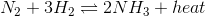By removing nitrogen or hydrogen gas, the system would shift to the left in order to create more reactants. If temperature is decreased, the system will create more heat by shifting to the right. Not only will this create more heat, but it will also create more ammonia, as they are both products of the reaction.

### Example Question #12 : Equilibrium Constant And Reaction Quotient

Consider the following generic reaction: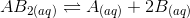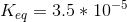If the equilibrium concentration of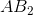is 1M, what is the equilibrium concentration of?

Possible Answers: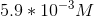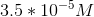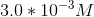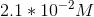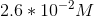Correct answer:Explanation:

We can set up the equilibrium expression by placing the products, raised to the power of their coefficients, in the numerator and reactants, raised to the power of their coefficients, in the denominator: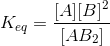We are given the value of the equilibrium constant and the equilibrium concentration of. Using stoichiometric coefficients, we can determine that a variable concentration,, ofwill be present at equilibrium, andofwill be present at equilibrium. Plugging in: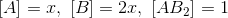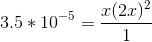Simplify: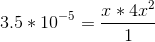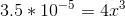Now we can solve for(which is the equilibrium concentration of, as we assigned) by dividing both sides by 4 and then taking the cube root.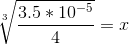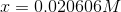Rounding to 2 sig figs (to match the two numbers we were given by the problem), we get: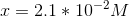### All High School Chemistry Resources